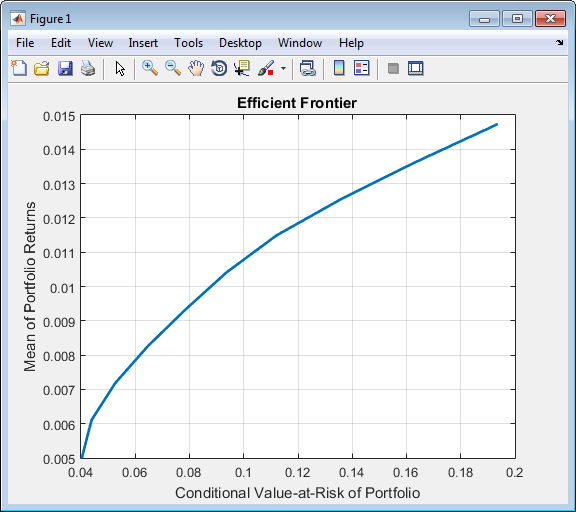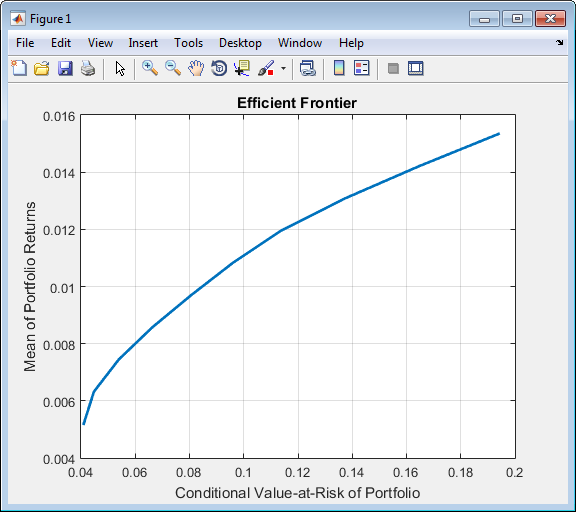## Creating the PortfolioCVaR Object

To create a fully specified CVaR portfolio optimization problem, instantiate the `PortfolioCVaR` object using `PortfolioCVaR`. For information on the workflow when using `PortfolioCVaR` objects, see PortfolioCVaR Object Workflow.

### Syntax

Use `PortfolioCVaR` to create an instance of an object of the `PortfolioCVaR` class. You can use `PortfolioCVaR` object in several ways. To set up a portfolio optimization problem in a `PortfolioCVaR` object, the simplest syntax is:

`p = PortfolioCVaR;`
This syntax creates a `PortfolioCVaR` object, `p`, such that all object properties are empty.

The `PortfolioCVaR` object also accepts collections of argument name-value pair arguments for properties and their values. The `PortfolioCVaR` object accepts inputs for public properties with the general syntax:

` p = PortfolioCVaR('property1', value1, 'property2', value2, ... );`

If a `PortfolioCVaR` object already exists, the syntax permits the first (and only the first argument) of `PortfolioCVaR` to be an existing object with subsequent argument name-value pair arguments for properties to be added or modified. For example, given an existing `PortfolioCVaR` object in `p`, the general syntax is:

`p = PortfolioCVaR(p, 'property1', value1, 'property2', value2, ... );`

Input argument names are not case-sensitive, but must be completely specified. In addition, several properties can be specified with alternative argument names (see Shortcuts for Property Names). The `PortfolioCVaR` object tries to detect problem dimensions from the inputs and, once set, subsequent inputs can undergo various scalar or matrix expansion operations that simplify the overall process to formulate a problem. In addition, a `PortfolioCVaR` object is a value object so that, given portfolio `p`, the following code creates two objects, `p` and `q`, that are distinct:

`q = PortfolioCVaR(p, ...)`

### PortfolioCVaR Problem Sufficiency

A CVaR portfolio optimization problem is completely specified with the `PortfolioCVaR` object if the following three conditions are met:

• You must specify a collection of asset returns or prices known as scenarios such that all scenarios are finite asset returns or prices. These scenarios are meant to be samples from the underlying probability distribution of asset returns. This condition can be satisfied by the `setScenarios` function or with several canned scenario simulation functions.

• The set of feasible portfolios must be a nonempty compact set, where a compact set is closed and bounded. You can satisfy this condition using an extensive collection of properties that define different types of constraints to form a set of feasible portfolios. Since such sets must be bounded, either explicit or implicit constraints can be imposed and several tools, such as the `estimateBounds` function, provide ways to ensure that your problem is properly formulated.

• You must specify a probability level to locate the level of tail loss above which the conditional value-at-risk is to be minimized. This condition can be satisfied by the `setProbabilityLevel` function.

Although the general sufficient conditions for CVaR portfolio optimization go beyond the first three conditions, the `PortfolioCVaR` object handles all these additional conditions.

### PortfolioCVaR Function Examples

If you create a `PortfolioCVaR` object, `p`, with no input arguments, you can display it using `disp`:

```p = PortfolioCVaR; disp(p); ```
```PortfolioCVaR with properties: BuyCost: [] SellCost: [] RiskFreeRate: [] ProbabilityLevel: [] Turnover: [] BuyTurnover: [] SellTurnover: [] NumScenarios: [] Name: [] NumAssets: [] AssetList: [] InitPort: [] AInequality: [] bInequality: [] AEquality: [] bEquality: [] LowerBound: [] UpperBound: [] LowerBudget: [] UpperBudget: [] GroupMatrix: [] LowerGroup: [] UpperGroup: [] GroupA: [] GroupB: [] LowerRatio: [] UpperRatio: []```

The approaches listed provide a way to set up a portfolio optimization problem with the `PortfolioCVaR` object. The custom set functions offer additional ways to set and modify collections of properties in the `PortfolioCVaR` object.

#### Using the PortfolioCVaR Function for a Single-Step Setup

You can use the `PortfolioCVaR` object to directly set up a “standard” portfolio optimization problem. Given scenarios of asset returns in the variable `AssetScenarios`, this problem is completely specified as follows:

```m = [ 0.05; 0.1; 0.12; 0.18 ]; C = [ 0.0064 0.00408 0.00192 0; 0.00408 0.0289 0.0204 0.0119; 0.00192 0.0204 0.0576 0.0336; 0 0.0119 0.0336 0.1225 ]; m = m/12; C = C/12; AssetScenarios = mvnrnd(m, C, 20000); p = PortfolioCVaR('Scenarios', AssetScenarios, ... 'LowerBound', 0, 'LowerBudget', 1, 'UpperBudget', 1, ... 'ProbabilityLevel', 0.95); ```
The `LowerBound` property value undergoes scalar expansion since `AssetScenarios` provides the dimensions of the problem.

You can use dot notation with the function `plotFrontier`.

`p.plotFrontier;`#### Using the PortfolioCVaR Function with a Sequence of Steps

An alternative way to accomplish the same task of setting up a “standard” CVaR portfolio optimization problem, given `AssetScenarios` variable is:

```m = [ 0.05; 0.1; 0.12; 0.18 ]; C = [ 0.0064 0.00408 0.00192 0; 0.00408 0.0289 0.0204 0.0119; 0.00192 0.0204 0.0576 0.0336; 0 0.0119 0.0336 0.1225 ]; m = m/12; C = C/12; AssetScenarios = mvnrnd(m, C, 20000); p = PortfolioCVaR; p = setScenarios(p, AssetScenarios); p = PortfolioCVaR(p, 'LowerBound', 0); p = PortfolioCVaR(p, 'LowerBudget', 1, 'UpperBudget', 1); p = setProbabilityLevel(p, 0.95); plotFrontier(p);```This way works because the calls to the are in this particular order. In this case, the call to initialize `AssetScenarios` provides the dimensions for the problem. If you were to do this step last, you would have to explicitly dimension the `LowerBound` property as follows:

```m = [ 0.05; 0.1; 0.12; 0.18 ]; C = [ 0.0064 0.00408 0.00192 0; 0.00408 0.0289 0.0204 0.0119; 0.00192 0.0204 0.0576 0.0336; 0 0.0119 0.0336 0.1225 ]; m = m/12; C = C/12; AssetScenarios = mvnrnd(m, C, 20000); p = PortfolioCVaR; p = PortfolioCVaR(p, 'LowerBound', zeros(size(m))); p = PortfolioCVaR(p, 'LowerBudget', 1, 'UpperBudget', 1); p = setProbabilityLevel(p, 0.95); p = setScenarios(p, AssetScenarios); ```

### Note

If you did not specify the size of `LowerBound` but, instead, input a scalar argument, the `PortfolioCVaR` object assumes that you are defining a single-asset problem and produces an error at the call to set asset scenarios with four assets.

#### Shortcuts for Property Names

The `PortfolioCVaR` object has shorter argument names that replace longer argument names associated with specific properties of the `PortfolioCVaR` object. For example, rather than enter `'ProbabilityLevel'`, the `PortfolioCVaR` object accepts the case-insensitive name `'plevel'` to set the `ProbabilityLevel` property in a `PortfolioCVaR` object. Every shorter argument name corresponds with a single property in the `PortfolioCVaR` object. The one exception is the alternative argument name `'budget'`, which signifies both the `LowerBudget` and `UpperBudget` properties. When `'budget'` is used, then the `LowerBudget` and `UpperBudget` properties are set to the same value to form an equality budget constraint.

Shortcuts for Property Names

Shortcut Argument Name

Equivalent Argument / Property Name

`ae`

`AEquality`

`ai`

`AInequality`

`assetnames` or `assets`

`AssetList`

`be`

`bEquality`

`bi`

`bInequality`

`budget`

`UpperBudget` and `LowerBudget`

`group`

`GroupMatrix`

`lb`

`LowerBound`

`n` or `num`

`NumAssets`

`level`, `problevel`, or `plevel`

`ProbabilityLevel`

`rfr`

`RiskFreeRate`

`scenario` or `assetscenarios`

`Scenarios`

`ub`

`UpperBound`

For example, this call to the `PortfolioCVaR` object uses these shortcuts for properties:

```m = [ 0.05; 0.1; 0.12; 0.18 ]; C = [ 0.0064 0.00408 0.00192 0; 0.00408 0.0289 0.0204 0.0119; 0.00192 0.0204 0.0576 0.0336; 0 0.0119 0.0336 0.1225 ]; m = m/12; C = C/12; AssetScenarios = mvnrnd(m, C, 20000); p = PortfolioCVaR('scenario', AssetScenarios, 'lb', 0, 'budget', 1, 'plevel', 0.95); plotFrontier(p); ```

#### Direct Setting of Portfolio Object Properties

Although not recommended, you can set properties directly using dot notation, however no error-checking is done on your inputs:

```m = [ 0.05; 0.1; 0.12; 0.18 ]; C = [ 0.0064 0.00408 0.00192 0; 0.00408 0.0289 0.0204 0.0119; 0.00192 0.0204 0.0576 0.0336; 0 0.0119 0.0336 0.1225 ]; m = m/12; C = C/12; AssetScenarios = mvnrnd(m, C, 20000); p = PortfolioCVaR; p = setScenarios(p, AssetScenarios); p.ProbabilityLevel = 0.95; p.LowerBudget = 1; p.UpperBudget = 1; p.LowerBound = zeros(size(m)); plotFrontier(p); ```

### Note

Scenarios cannot be assigned directly using dot notation to a `PortfolioCVaR` object. Scenarios must always be set through either the `PortfolioCVaR` object, the `setScenarios` function, or any of the scenario simulation functions.